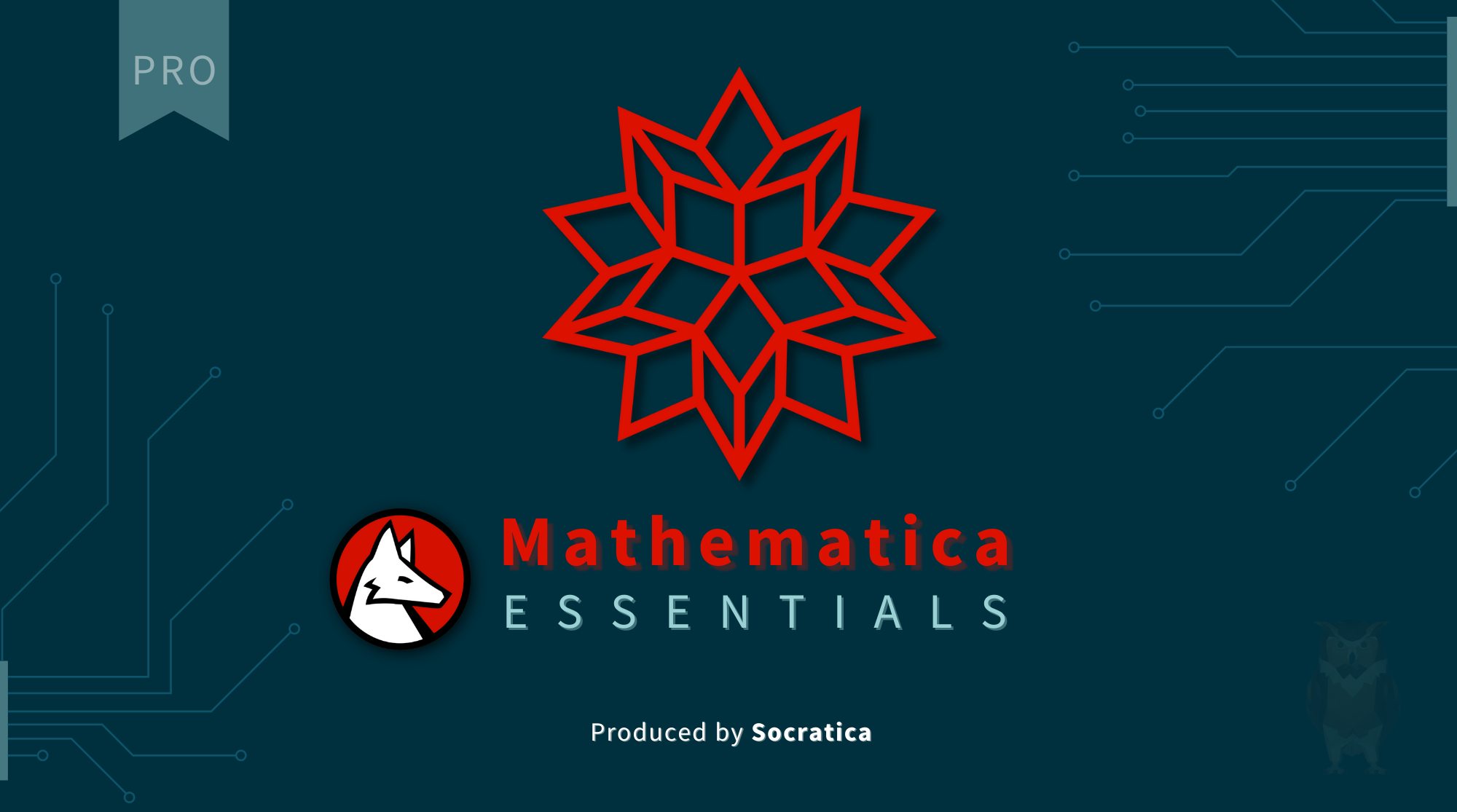# Mathematica

## Course Overview

Mathematica Essentials is a Pro Course from Socratica.

You can purchase the course on Vimeo at https://vimeo.com/ondemand/mathematica

The name Mathematica may sound like the program is just for mathematics; but it can be used in ANY computational field: financial analysis, machine learning, data visualization, bioinformatics, speech recognition, and much, much more.

Our Mathematica Essentials course includes the following lessons:

1. Introduction & Overview to Mathematica and the Wolfram Language
2. First Notebook - Mathematica Essentials
3. Mathematica as a Calculator
4. Numerical Types
5. Cells
6. Mathematica Documentation
7. Functions
8. Custom Functions
9. Lists
10. Expressions - The Building Block of Everything
11. Introduction to Plots
12. 2D Graphics - Primitives & Directives
13. 3D Graphics
14. Import & Export
15. Solving Equations in Mathematica
16. Polynomial Equations
17. Derivatives
18. Integrals
19. Entities in Mathematica
20. Modular Arithmetic

In addition to the 20 lessons above, our course also has some bonus features:

• Watch the Cursor
• Using Stylesheets
• Reducing Square Brackets
• Text Sizing
• Exporting Plots & Graphics

Purchase today on Vimeo at https://vimeo.com/ondemand/mathematica## ResourcesMathematica Essentials
A comprehensive first course in Mathematica from Socratica.An Elementary Introduction to the Wolfram Language
A free, online book to help beginner's learn the Wolfram Language.Wolfram Language
Documentation for Mathematica and the Wolfram Language.